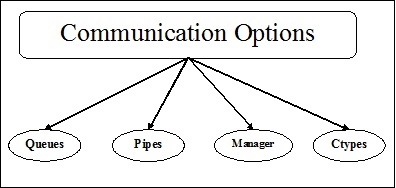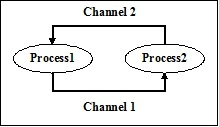# Processes Intercommunication

Process intercommunication means the exchange of data between processes. It is necessary to exchange the data between processes for the development of parallel application. Following diagram shows the various communication mechanisms for synchronization between multiple sub processes −## Various Communication Mechanisms

In this section, we will learn about the various communication mechanisms. The mechanisms are described below −

### Queues

Queues can be used with multi-process programs. The Queue class of multiprocessing module is similar to the Queue.Queue class. Hence, the same API can be used. Multiprocessing.Queue provides us a thread and process safe FIFO (first-in first-out) mechanism of communication between processes.

### Example

Following is a simple example taken from python official docs on multiprocessing to understand the concept of Queue class of multiprocessing.

```from multiprocessing import Process, Queue
import queue
import random
def f(q):
q.put([42, None, 'hello'])
def main():
q = Queue()
p = Process(target = f, args = (q,))
p.start()
print (q.get())
if __name__ == '__main__':
main()```

### Output

```>[42, None, 'hello']
```

### Pipes

It is a data structure, which is used to communicate between processes in multi-process programs. The Pipe() function returns a pair of connection objects connected by a pipe which by default is duplex(two way). It works in the following manner −

• It returns a pair of connection objects that represent the two ends of pipe.

• Every object has two methods – send() and recv(), to communicate between processes.

### Example

Following is a simple example taken from python official docs on multiprocessing to understand the concept of Pipe() function of multiprocessing.

```from multiprocessing import Process, Pipe

def f(conn):
conn.send([42, None, 'hello'])
conn.close()

if __name__ == '__main__':
parent_conn, child_conn = Pipe()
p = Process(target = f, args = (child_conn,))
p.start()
print (parent_conn.recv())
p.join()```

### Output

```>[42, None, 'hello']
```

### Manager

Manager is a class of multiprocessing module that provides a way to coordinate shared information between all its users. A manager object controls a server process, which manages shared objects and allows other processes to manipulate them. In other words, managers provide a way to create data that can be shared between different processes. Following are the different properties of manager object −

• The main property of manager is to control a server process, which manages the shared objects.

• Another important property is to update all the shared objects when any process modifies it.

### Example

Following is an example which uses the manager object for creating a list record in server process and then adding a new record in that list.

```import multiprocessing

def print_records(records):
for record in records:
print("Name: {0}\nScore: {1}\n".format(record, record))

def insert_record(record, records):
records.append(record)

if __name__ == '__main__':
with multiprocessing.Manager() as manager:

records = manager.list([('Computers', 1), ('Histoty', 5), ('Hindi',9)])
new_record = ('English', 3)

p1 = multiprocessing.Process(target = insert_record, args = (new_record, records))
p2 = multiprocessing.Process(target = print_records, args = (records,))
p1.start()
p1.join()
p2.start()
p2.join()```

### Output

```>A New record is added

Name: Computers
Score: 1

Name: Histoty
Score: 5

Name: Hindi
Score: 9

Name: English
Score: 3
```

### Concept of Namespaces in Manager

Manager Class comes with the concept of namespaces, which is a quick way method for sharing several attributes across multiple processes. Namespaces do not feature any public method, which can be called, but they have writable attributes.

### Example

The following Python script example helps us utilize namespaces for sharing data across main process and child process −

```import multiprocessing

def Mng_NaSp(using_ns):

using_ns.x +=5
using_ns.y *= 10

if __name__ == '__main__':
manager = multiprocessing.Manager()
using_ns = manager.Namespace()
using_ns.x = 1
using_ns.y = 1

print ('before', using_ns)
p = multiprocessing.Process(target = Mng_NaSp, args = (using_ns,))
p.start()
p.join()
print ('after', using_ns)```

### Output

```>before Namespace(x = 1, y = 1)
after Namespace(x = 6, y = 10)
```

## Ctypes-Array and Value

Multiprocessing module provides Array and Value objects for storing the data in a shared memory map. Array is a ctypes array allocated from shared memory and Value is a ctypes object allocated from shared memory.

To being with, import Process, Value, Array from multiprocessing.

### Example

Following Python script is an example taken from python docs to utilize Ctypes Array and Value for sharing some data between processes.

```def f(n, a):
n.value = 3.1415927
for i in range(len(a)):
a[i] = -a[i]

if __name__ == '__main__':
num = Value('d', 0.0)
arr = Array('i', range(10))

p = Process(target = f, args = (num, arr))
p.start()
p.join()
print (num.value)
print (arr[:])```

### Output

```>3.1415927
[0, -1, -2, -3, -4, -5, -6, -7, -8, -9]
```

## Communicating Sequential Processes (CSP)

CSP is used to illustrate the interaction of systems with other systems featuring concurrent models. CSP is a framework for writing concurrent or program via message passing and hence it is effective for describing concurrency.

## Python library – PyCSP

For implementing core primitives found in CSP, Python has a library called PyCSP. It keeps the implementation very short and readable so that it can be understood very easily. Following is the basic process network of PyCSP −In the above PyCSP process network, there are two processes – Process1 and Process 2. These processes communicate by passing messages through two channels – channel 1 and channel 2.

### Installing PyCSP

With the help of following command, we can install Python library PyCSP −

`pip install PyCSP`

### Example

Following Python script is a simple example for running two processes in parallel to each other. It is done with the help of the PyCSP python libabary −

```from pycsp.parallel import *
import time
@process
def P1():
time.sleep(1)
print('P1 exiting')
@process
def P2():
time.sleep(1)
print('P2 exiting')
def main():
Parallel(P1(), P2())
print('Terminating')
if __name__ == '__main__':
main()```

In the above script, two functions namely P1 and P2 have been created and then decorated with @process for converting them into processes.

### Output

```>P2 exiting
P1 exiting
Terminating
```